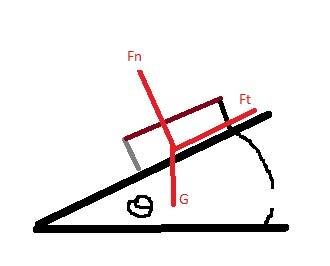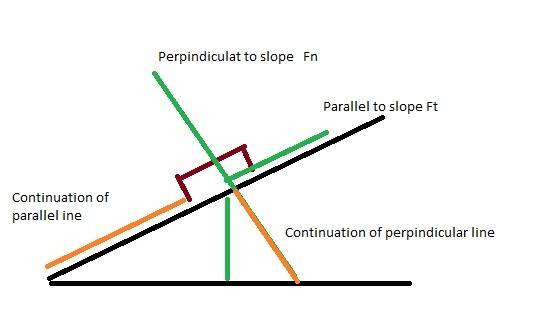# How to find forces acting on object on a slope?

urbano

## Homework Statement

an object is at rest on a slope at angle of σ

Using trigonometry, solve the forces acting at Ft and FnSoh
Cah
Toa

## The Attempt at a Solution

sin σ = G/Ft
sin σ /Ft = G
G/ sin σ = Ft (my final answer)

Book says correct answer is Ft = G sinσ

Fn = ??? I wanst even sure how to strat this using σ

The book says the correct answer is Fn = G cosσ

## Answers and Replies

Staff Emeritus
The force G can be resolved into 2 components: one which is normal to the slope (the exact opposite to Fn), and one which is parallel to the slope (the exact opposite to Ft).

Draw the triangle of forces showing how G equals the vector sum of these two forces.

urbano
edited...I'll be back

Last edited:
urbano
Thanks NascentOxygen. Unfortunately I dont quite get why the answers are what they are. I have drawn myself a new triangle which shows the two vector forces but I still don't understand where the answers have come from.

Especially Fn = G cosσ. I thought cos in basic terms meant adjacent/hypothenuse . So if the sloped line is the hypothenuse and the bottom line is the adjacent once I draw the line which is exact opposite to Fn I now no longer have a right angle triangle.....arghh!! I'm just so confused...

Staff Emeritus

urbano
here is my latest diagramI'm now starting to think Soh Cah Toa isn't actually relevant there ?

Fn = G cosσ Ft = Gσ

I'm unsure where these two formulas/equations have come from and why Soh Cah Toa isn't relevant here...

I must be missing something fundamental

Staff Emeritus